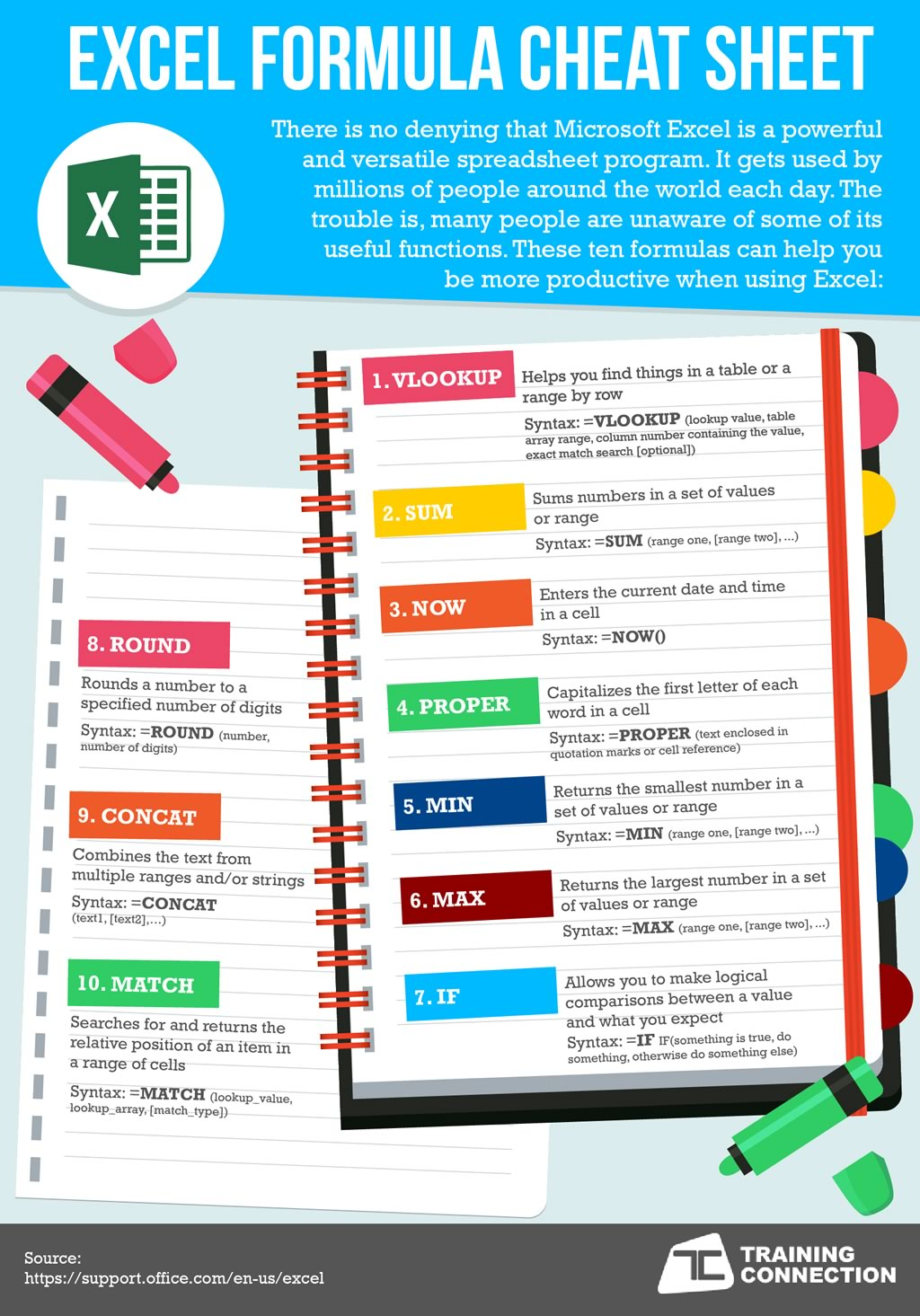888.815.0604
Home / Resources / Microsoft Office / Excel / Formula Cheatsheet

# Excel Formula Cheatsheet## Excel Formula Cheat Sheet

There is no denying that Microsoft Excel is a powerful and versatile spreadsheet program. It gets used by millions of people around the world each day. The trouble is, many people are unaware of some of its useful functions. These ten formulas can help you be more productive when using Excel:

1. VLOOKUP
• Helps you find things in a table or a range by row
• Syntax: =VLOOKUP(lookup value, table array range, column number containing the value, exact match search [optional])

2. SUM
• Sums numbers in a set of values or range
• Syntax: =SUM(range one, [range two], ...)

3. NOW
• Enters the current date and time in a cell
• Syntax: =NOW()

4. PROPER
• Capitalizes the first letter of each word in a cell
• Syntax: =PROPER(text enclosed in quotation marks or cell reference)

5. MIN
• Returns the smallest number in a set of values or range
• Syntax: =MIN(range one, [range two], ...)

6. MAX
• Returns the largest number in a set of values or range
• Syntax: =MAX(range one, [range two], ...)

7. IF
• Allows you to make logical comparisons between a value and what you expect
• Syntax: =IF(something is true, do something, otherwise do something else)

8. ROUND
• Rounds a number to a specified number of digits
• Syntax: =ROUND(number, number of digits)

9. CONCAT
• Combines the text from multiple ranges and/or strings
• Syntax: =CONCAT(text1, [text2],…)

10. MATCH
• Searches for and returns the relative position of an item in a range of cells
• Syntax: =MATCH(lookup_value, lookup_array, [match_type])

Source:

## Our Excel training solutions

We run traditional face-to-face Excel classes for all levels of users in Chicago and Los Angeles. We also deliver onsite Excel training across the country. Or view our Excel course outlines at the links below.